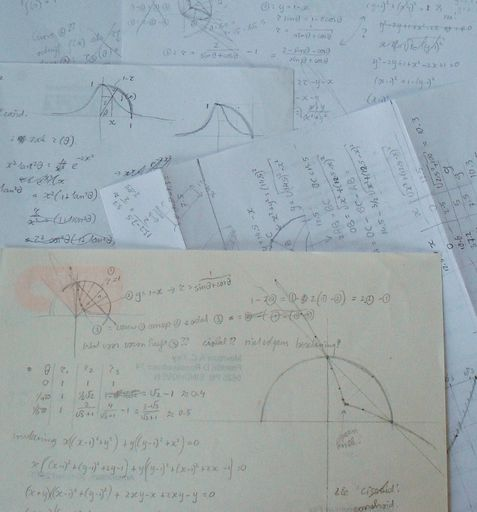I like to apply math for my bookfolding designs, and not only in obvious ways, as in 'Sine and cosine', 'Fractal' or 'Infinity'. Most of the math is pretty basic, but occasionally I stumble upon something slightly more advanced, such as the conchoid curves of Nicomedes, or spline curves. I'll start with the basic stuff:

I like to figure out every detail of my designs before I start folding, such that cutting is avoided as much as possible. The depth of the fold determines how far the pages are spread out. For instance, in 'Don't close me', the bottom folds have to be deeper because they are pressed down by the pages on top; the depth of the fold should vary linearly with the page number.

Angles are important; if an angle is too acute then it can't be folded well, so I need to keep that in mind too while determining the depth of my folds. The number of pages determines the amount of detail that will show, which is important in works like 'Reading'.

In 'Ring', half the folds stick out and the other half is folded in. But the surface between is curved, so the distances are different. I calculated how far each fold should go such that they do form a circle. Also, I took perspective in account: the inward folds are narrower than the outward ones.

In 'Book end', I made the figurine appear at the bottom of the book by folding the pages inward. But they can't fold further than to the center without cutting, and not much less than to the middle. So I made the design such that the figurine remains between these bounds.

I became intrigued with the mathematics of displaying the bottom of the book (see 'Curves', 'Bell curve', 'Circles' and 'Conchoid'). The pages fan out radially, and one can make all sorts of zig-zag folds. While designing 'Bell curve', I noticed that the ends of the inward folded pages formed another interesting curve. It turns out that the family of such curves has been studied ca. 200 B.C. by Nicomedes. He called them conchoids, because they reminded him of shells.

Here is the math: We start with a fixed point O, a curve, and a circle around O of radius r. Draw a straight line through O that intersects the curve in A. Mark point B on this line, such that AB = r (in fact there are two such points). Do this for every line through O; then all marked points together form the conchoid of the curve. The conchoid consists of two branches. For one of the branches, OB = r + OA, and for the other OB = r - OA.

Back to bookfolding: look at the bottom of the book. The point O is at the spine, r is the page width plus a bit of room near the spine, so that the ends of the pages form the circle. Every page displays as a straight line through O. Now choose a curve that nicely intersects the circle, for example the straight line I chose for 'Conchoid'. Pick a page, locate point A where it intersects the curve, and fold the page inward at that point. The page end is now at a point B, closer to the spine. We didn't change the width of the pages, so we have OA + AB = r. Then OB = r - 2OA. So we created one branch of a conchoid, not Nicomedes' conchoid of the curve we chose, but of a related curve. In 'Conchoid' for example, it is the line parallel to the visible one, but at twice the distance from O.

Gradually I am becoming more interested into curving and bending the book pages rather than folding. In 2019 I decided to cut along the margin of the pages and pull the half-attached paper strips in various ways. They then bend into all sorts of aesthetically pleasing curves - see 'Dance' and 'Feathers'. These curves are naturally determined by the property of the paper strips to settle in a state of minimal strain. Such curves are known as 'splines'. They are usually approximated by polynomials, and calculated numerically, so I have no exact formulas for mine.

I do my calculations by hand, with pencil and paper. I don't even like to use a calculator. If a problem can't be solved analytically, I solve it graphically using a pencil, calipers and a ruler, rather than using a computer. It's not that I don't know how; during my math research years I wrote many programs. But I experienced that relying on the computer leads to neglecting the classical and elegant geometry and analysis tools and skills, which much better accompany a work of art. I actually rediscovered some ancient greek mathematics, from the age when there was no difference between being a mathematician or an artist.

To me, doing the math by hand is as much part of the artwork as the folding itself.